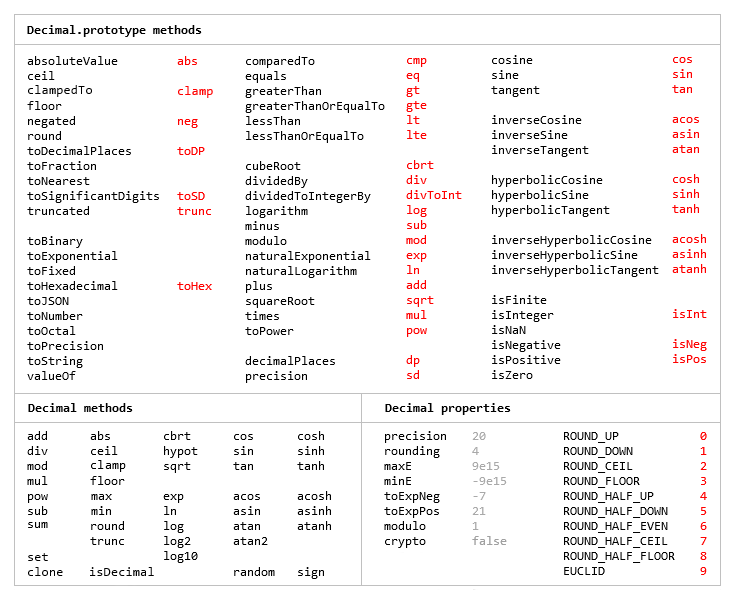# Library of The Week: decimal.js

Every child can tell you that 1 + 2 = 3. But what is 0.1 + 0.2? As developer, you are doubtless aware that the result when using floating-point arithmetic isn't exactly 0.3 but rather 0.30000000000000004.

That's good enough for many applications, but in some cases we need to be more precise. A typical real-life example: imagine you are calculating the total price of an item that costs \$168.70 with 15% sales tax. If you round up to the nearest cent (a common approach for many currencies), you will get 194.01. But using floats, 168.7 * 1.15 is 194.00499999999997, which rounds down to 194.00. This might be of interest to Richard Pryor but it won't make your accountant very happy.

To deal with this, programming languages usually provide arbitrary precision decimal types, often as part of the their standard runtime library. We have BigDecimal in Java, decimal in Python and GMP library for C/C++. As for JavaScript, we can use—wait for it!—decimal.js.

Here's how to deal with the aforementioned calculation using decimal.js:

``````> new Decimal('168.70').times('1.15').toFixed(2)
'194.01'
``````

Which is exactly what we want.

Here's a handy guide to the full decimal.js API: